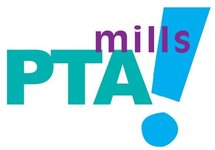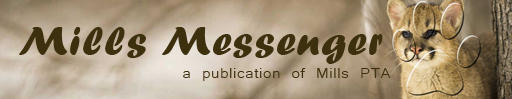## PTA Message

 table div table+table+table+table div table{width:100%;padding:0}table div table+table+table+table div table img{width:96.23%;padding:0;float:none}table div table+table+table+table div table td{width:100%;padding:0 1.88% 18px}/* styles */table div table+table+table+table+table div table,table.module-4{width:71.7%;float:left;padding:0}table div table+table+table+table+table div table a{border:0 none;text-decoration:none}table div table+table+table+table+table div table img{width:100%!important;border:0 none;text-decoration:none}table div table+table+table+table+table div table td{width:100%;padding:0 20px 20px 0}/* styles */ BUY YOUR YEARBOOK TODAY! THE FIRST 50 BUYERS RECEIVE FREE PLASTIC YEARBOOK COVERS. Purchase online at www.balfour.com for only \$25
 table div table+table+table+table+table+table+table div table{width:100%;padding:0}table div table+table+table+table+table+table+table div table img{width:96.23%;padding:0;float:none}table div table+table+table+table+table+table+table div table td{width:100%;padding:0 1.88% 18px}/* styles */## Hello World - Fall Virtual Computer Science Program

Hello World computer science programs are designed by PhDs from Harvard, MIT, and Stanford University. High school alumni students have developed impressive portfolios as a part of their applications to Tier 1 colleges. Course topics include Web Development, Game Engineering, Hacking Minecraft, iOS Mobile App Development, and Music Programming. Classes are taught with a 5:1 student to engineering instructor ratio, and all sessions are led by professional engineers. Learn more here: www.helloworldstudio.org/hello-world-institute

 table div table+table+table+table+table+table+table+table+table+table div table{width:100%;padding:0}table div table+table+table+table+table+table+table+table+table+table div table img{width:96.23%;padding:0;float:none}table div table+table+table+table+table+table+table+table+table+table div table td{width:100%;padding:0 1.88% 18px}/* styles */table.module-10{width:57.74%;padding:0}table div table+table+table+table+table+table+table+table+table+table+table div table{width:57.74%;float:none;margin-left:auto;margin-right:auto;padding:0}table div table+table+table+table+table+table+table+table+table+table+table div table a{border:0 none;text-decoration:none}table div table+table+table+table+table+table+table+table+table+table+table div table img{width:100%!important;border:0 none;text-decoration:none}table div table+table+table+table+table+table+table+table+table+table+table div table td{width:100%;padding:0}/* styles */
 table div table+table+table+table+table+table+table+table+table+table+table+table div table{width:100%;padding:0}table div table+table+table+table+table+table+table+table+table+table+table+table div table img{width:96.23%;padding:0;float:none}table div table+table+table+table+table+table+table+table+table+table+table+table div table td{width:100%;padding:0 1.88% 18px}/* styles */## Need to get your message out?

 /* styles */ Submit info to the Mills Messenger. Want to see the Mills Calendar on your Google calendar? Add Mills Calendar to my Google Calendar. Have a friend who isn't getting the Messenger but wants to? Send them this link to sign up: https://madmimi.com/signups/join/7059
 table div table+table+table+table+table+table+table+table+table+table+table+table+table+table+table div table{width:100%;padding:0}table div table+table+table+table+table+table+table+table+table+table+table+table+table+table+table div table img{width:96.23%;padding:0;float:none}table div table+table+table+table+table+table+table+table+table+table+table+table+table+table+table div table td{width:100%;padding:0 1.88% 18px}/* styles */